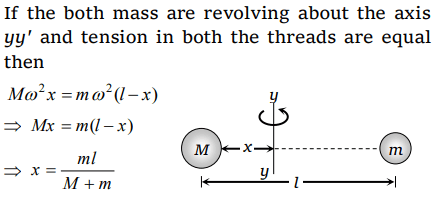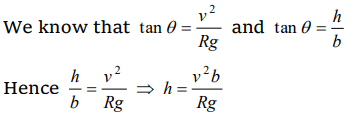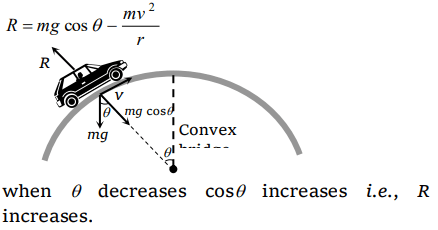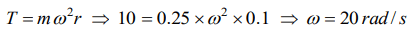## Motion in a Plane Questions and Answers Part-5

1. A particle moves in a circular orbit under the action of a central attractive force inversely proportional to the distance 'r' . The speed of the particle is
a) Proportional to $r^{2}$
b) Independent of r
c) Proportional to r
d) Proportional to$\frac{1}{r}$

Explanation:2. Two masses M and m are attached to a vertical axis by weightless threads of combined length l . They are set in rotational motion in a horizontal plane about this axis with constant angular velocity $\omega$ . If the tensions in the threads are the same during motion, the distance of M from the axis is
a) $\frac{Ml}{M+m}$
b) $\frac{ml}{M+m}$
c) $\frac{M+m}{M}l$
d) $\frac{M+m}{m}l$

Explanation:3. A boy on a cycle pedals around a circle of 20 metres radius at a speed of 20 metres/sec. The combined mass of the boy and the cycle is 90 kg. The angle that the cycle makes with the vertical so that it may not fall is $\left(g=9.8 m\diagup sec^{2}\right)$
a) $60.25^{\circ}$
b) $63.90^{\circ}$
c) $26.12^{\circ}$
d) $30.00^{\circ}$

Explanation:4. The average acceleration vector for a particle having a uniform circular motion is
a) A constant vector of magnitud$\frac{v^{2}}{r}$
b) A vector of magnitude $\frac{v^{2}}{r}$ directed normal to the plane of the given uniform circular motion
c) Equal to the instantaneous acceleration vector at the start of the motion
d) A null vector

Explanation: In complete revolution change in velocity becomes zero so average acceleration will be zero

5. Radius of the curved road on national highway is R . Width of the road is b . The outer edge of the road is raised by h with respect to inner edge so that a car with velocity v can pass safe over it. The value of h is
a) $\frac{v^{2}b}{Rg}$
b) $\frac{v}{Rgb}$
c) $\frac{v^{2}R}{g}$
d) $\frac{v^{2}b}{R}$

Explanation:6. When a particle moves in a uniform circular motion. It has
b) Tangential velocity and radial acceleration
c) Tangential velocity and tangential acceleration
d) Radial velocity and tangential acceleration

Explanation: Tangential velocity and radial acceleration

7. A motorcycle is going on an overbridge of radius R . The driver maintains a constant speed. As the motorcycle is ascending on the overbridge, the normal force on it
a) Increases
b) Decreases
c) Remains the same
d) Fluctuates

Explanation:8. A mass of 2 kg is whirled in a horizontal circle by means of a string at an initial speed of 5 revolutions per minute. Keeping the radius constant the tension in the string is doubled. The new speed is nearly
a) 14 rpm
b) 10 rpm
c) 2.25 rpm
d) 7 rpm

Explanation:9. The magnitude of the centripetal force acting on a body of mass m executing uniform motion in a circle of radius r with speed v is
a) mvr
b) $mv^{2}\diagup r$
c) $v\diagup r^{2}m$
d) $v\diagup r m$

Explanation: $mv^{2}\diagup r$